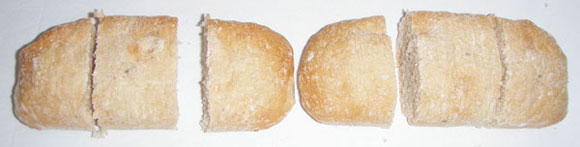Home > Fractions > Good teaching > Dividing > Sharing as division

# Sharing as division

Students model problems that require them to cut the items into fractional parts to carry out the sharing process.

For example, share two loaves equally between three people. Each person receives two-thirds of a loaf: 2 ÷ 3 = $$\frac{2}{3}$$.Two loaves shared evenly between three people.

Sharing problems build the foundation for understanding fractions as an expression of division, as in $$\frac{2}{3}$$ = 2 ÷ 3.

Sharing problems also provide the important opportunity for students to experience the process of re-unitising. Re-unitising occurs when the size of the fraction being used is changed to suit the needs of the problem.

For example, in the problem of three loaves shared equally between four people, the first step could be to halve the loaves. This gives each person one half of a loaf and uses two of the loaves.

The third loaf can then be cut into four equal parts and the sharing continued. So each person gets one half and one quarter, making three quarters of a loaf each. Watch the video The Sharing Process.

Sharing diagrams such as the one illustrated above should feature in student recording of their solution strategies.

## Dividing pancakes

Students physically model problems that require them to cut the items into fractional parts to carry out the sharing process.